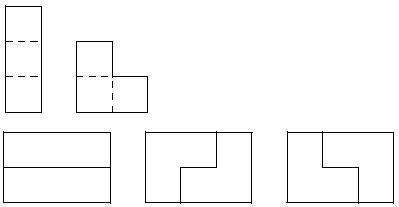Competitions

# Triomino

In how many ways can you tile a 2 × n rectangle with triominoes? A triomino is a geometric shape made from three squares joined along complete edges. There are only two possible triominoes:For example, you can tile a 2 × 3 rectangle only in 3 different ways. As the answer can be quite big, you just need to find the number of ways modulo 106.

Input

The first line contains the number of test cases t (1 ≤ t ≤ 100). Each of the following t lines contains the value of n (0 < n < 109).

Output

For each test case print in a separate line a number of ways you can tile a 2 × n rectangle. Print the answer modulo 106.

Time limit 1 second
Memory limit 64 MiB
Input example #1
3
3
4
6

Output example #1
3
0
11